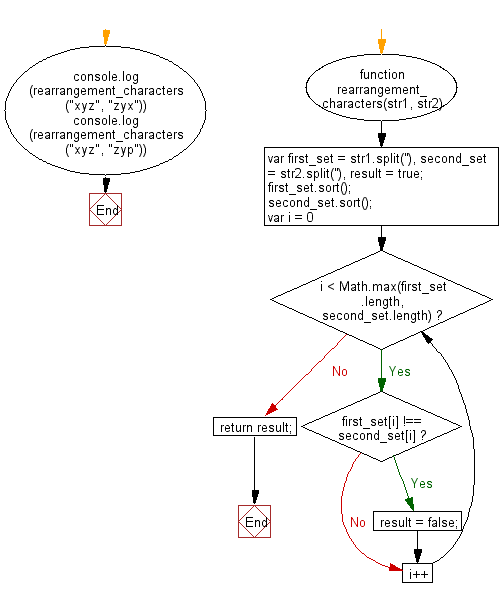# JavaScript: Check whether a given fraction is proper or not

## JavaScript Basic: Exercise-133 with Solution

Write a JavaScript program to check whether a given fraction is proper or not.

Note: There are two types of common fractions, proper or improper. When the numerator and the denominator are both positive, the fraction is called proper if the numerator is less than the denominator, and improper otherwise.

Sample Solution:

HTML Code:

``````<!DOCTYPE html>
<html>
<meta charset="utf-8">
<meta name="viewport" content="width=device-width">
<title>Check whether a given fraction is proper or not</title>
<body>

</body>
</html>
```
```

JavaScript Code:

``````function proper_improper_test(num) {
return Math.abs(num / num) < 1
? "Proper fraction."
: "Improper fraction.";
}
console.log(proper_improper_test([12, 300]));
console.log(proper_improper_test([2, 4]));
console.log(proper_improper_test([103, 3]));
console.log(proper_improper_test([104, 2]));
console.log(proper_improper_test([5, 40]));
``````

Sample Output:

```Proper fraction.
Proper fraction.
Improper fraction.
Improper fraction.
Proper fraction.
```

Flowchart:ES6 Version:

``````function proper_improper_test(num) {
return Math.abs(num / num) < 1
? "Proper fraction."
: "Improper fraction.";
}
console.log(proper_improper_test([12, 300]));
console.log(proper_improper_test([2, 4]));
console.log(proper_improper_test([103, 3]));
console.log(proper_improper_test([104, 2]));
console.log(proper_improper_test([5, 40]));
``````

Live Demo:

See the Pen javascript-basic-exercise-133 by w3resource (@w3resource) on CodePen.

Improve this sample solution and post your code through Disqus

What is the difficulty level of this exercise?

Test your Programming skills with w3resource's quiz.

﻿

## JavaScript: Tips of the Day

Converts a string to title case

Example:

```const toTitleCase = str =>
str
.match(/[A-Z]{2,}(?=[A-Z][a-z]+[0-9]*|\b)|[A-Z]?[a-z]+[0-9]*|[A-Z]|[0-9]+/g)
.map(x => x.charAt(0).toUpperCase() + x.slice(1))
.join(' ');
console.log(toTitleCase('some_database_field_name')); // 'Some Database Field Name'
console.log(toTitleCase('Some label that needs to be title-cased')); // 'Some Label That Needs To Be Title Cased'
console.log(toTitleCase('some-package-name')); // 'Some Package Name'
console.log(toTitleCase('some-mixed_string with spaces_underscores-and-hyphens')); // 'Some Mixed String With Spaces Underscores And Hyphens'
```

Output:

```"Some Database Field Name"
"Some Label That Needs To Be Title Cased"
"Some Package Name"
"Some Mixed String With Spaces Underscores And Hyphens"
```